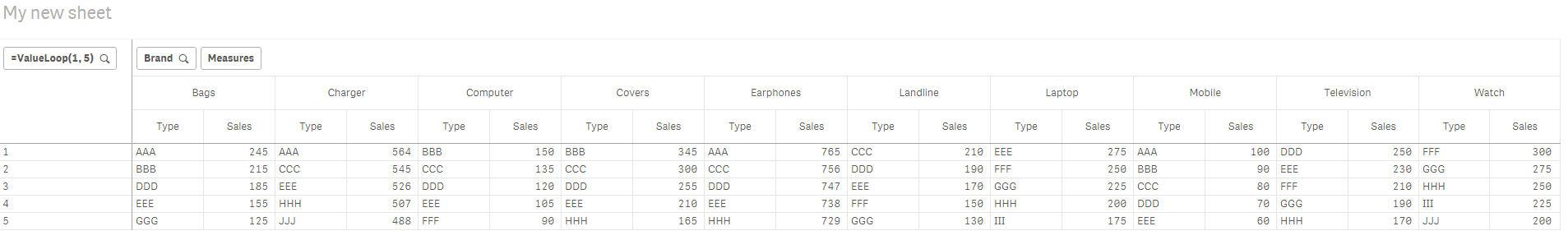# New to Qlik Sense

Discussion board where members can get started with Qlik Sense.

Announcements
Coming your way, the Qlik Data Revolution Virtual Summit. October 27-29. REGISTER
cancel
Showing results for
Did you mean:
HighlightedContributor III

## Top Ranks based on sales w.r.t Brands and Type in Pivot Table in Qlik Sense.

Hello Qlik Experts,

I have the following data in a table format and want to find out the Top Ranks based on Sales w.r.t Brands and Type in Pivot Table.

I require the output in a Pivot Table.

I am attaching a sample data Below in Excel format, where in I have to find the Top 5 Ranks of each Brands based on their Types and Sales.

The Input Data and the Output format is displayed in the Excel Workbook in different sheets.

1 Solution

Accepted SolutionsMVP

Bottom chart can be the other way you can do this... but then it looks different from what you needDimensions

Type

Brand

Expression

Aggr(If(Rank(Sum(Sales)) < 6, Sum(Sales)), Brand, Type)

7 Replies
HighlightedPartner

HI,

Satish find the attached QliksenseApp

may it full fill your requirement. I used below expression for the dimension.

=Aggr(If(Rank(SUM(Sales)) <= 5, Type), Brand,Type)

HighlightedMVP

This?Dimensions

=ValueLoop(1, 5)

Brand

Expressions

Pick(ValueLoop(1, 5),

Aggr(NODISTINCT If(Rank(Sum(Sales)) = 1, Type), Brand, Type),

Aggr(NODISTINCT If(Rank(Sum(Sales)) = 2, Type), Brand, Type),

Aggr(NODISTINCT If(Rank(Sum(Sales)) = 3, Type), Brand, Type),

Aggr(NODISTINCT If(Rank(Sum(Sales)) = 4, Type), Brand, Type),

Aggr(NODISTINCT If(Rank(Sum(Sales)) = 5, Type), Brand, Type))

Pick(ValueLoop(1, 5),

Aggr(NODISTINCT If(Rank(Sum(Sales)) = 1, Sum(Sales)), Brand, Type),

Aggr(NODISTINCT If(Rank(Sum(Sales)) = 2, Sum(Sales)), Brand, Type),

Aggr(NODISTINCT If(Rank(Sum(Sales)) = 3, Sum(Sales)), Brand, Type),

Aggr(NODISTINCT If(Rank(Sum(Sales)) = 4, Sum(Sales)), Brand, Type),

Aggr(NODISTINCT If(Rank(Sum(Sales)) = 5, Sum(Sales)), Brand, Type))

HighlightedContributor III

Thanks Sunny,

However if I have to find the Values for Top 10, 20, 50 and so on, will I have to Have the Same equation in a longer format incrementing the Numbers.

HighlightedMVP

Well, I am not sure if there is another way... did you check out what arunaerra‌ posted... I am not sure what they have... but may be that works better for you...

HighlightedContributor III

Yes I checked what Aruna posted, however That doesn't solve my problem.MVP

Bottom chart can be the other way you can do this... but then it looks different from what you needDimensions

Type

Brand

Expression

Aggr(If(Rank(Sum(Sales)) < 6, Sum(Sales)), Brand, Type)

HighlightedContributor III

Thanks a lot Sunny